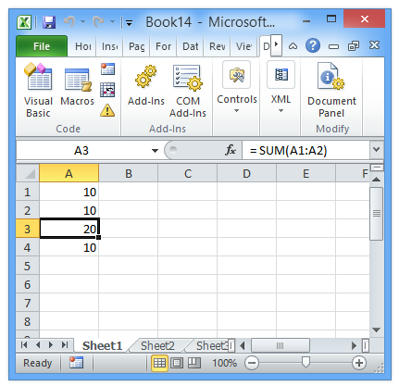# Excel ActiveCell.FormulaR1C1 error

hello all, I am making an application in excel but when I use referecias to other cells or operations between cells mark me an error

``````Dim excel As New ExcelApplication
Dim book As ExcelWorkbook

excel.Visible = True
excel.Range("A1","A1").Select_
excel.ActiveCell.FormulaR1C1=10
excel.ActiveCell.Offset(1,0).Select_
excel.ActiveCell.FormulaR1C1=10
excel.ActiveCell.Offset(1,0).Select_
excel.ActiveCell.FormulaR1C1="=sum(A1:A2)"
excel.ActiveCell.Offset(1,0).Select_
excel.ActiveCell.FormulaR1C1"=R[-3]C+R[-2]C" ' here is the problem send OLEException
excel.ActiveCell.FormulaR1C1= "=RC[-1]" ' this line too  have error send OLEException``````

What does the OLEException message tell you? If you’re running this code in the debugger and you’ve hit this exception, just resume the app and you should see an Exception dialog with some kind of message.

Hello Vania,

Unfortunately the FormulaR1C1 command does not currently exist in the code. As an alternative, the following code can be used:

[code] Dim excel As New ExcelApplication
Dim book As ExcelWorkbook

excel.Visible = True
excel.Range(“A1”).Value = 10
excel.Range(“A2”).Value = 10
excel.Range(“A3”).Value = “=sum(A1:A2)”
[/code]

Happy to help,

Eugene

My apologies, the command does exist and here is code which provides no errors (there is a logical error though).

[code] Dim excel As New ExcelApplication
Dim book As ExcelWorkbook

excel.Visible = True
excel.Range(“A1”,“A1”).Select_
excel.ActiveCell.FormulaR1C1=10
excel.ActiveCell.Offset(1,0).Select_
excel.ActiveCell.FormulaR1C1=10
excel.ActiveCell.Offset(1,0).Select_
excel.ActiveCell.FormulaR1C1="=sum(A1:A2)"
excel.ActiveCell.Offset(1,0).Select_
excel.ActiveCell.FormulaR1C1 = “=R[-3]C+R[-2]C” 'It works now
excel.ActiveCell.FormulaR1C1 = “=RC[-1]” 'This works too[/code]

Let me know what you are trying to do and we can work on an example together.Sincerely,

Eugene

hi this show when i run program
run in xojo and realstudioHello Vania,

Thanks for your reply. Please check to see if the second last line of code has been changed:

``excel.ActiveCell.FormulaR1C1"=R[-3]C+R[-2]C" ' here is the problem send OLEException``

Should be:

``excel.ActiveCell.FormulaR1C1 = "=R[-3]C+R[-2]C" 'It works now``

An equal sign (=) should be added before the quotation marks ("). (Cambie el cdigo con el error de la lnea para incluir un signo igual. Un signo igual no se encuentra.)

Let me know if this works for you.

Sincerely,

Eugene

hello

`````` Dim excel As New ExcelApplication
Dim book As ExcelWorkbook

excel.Visible = True
excel.Range("A1","A1").Select_
excel.ActiveCell.FormulaR1C1=10
excel.ActiveCell.Offset(1,0).Select_
excel.ActiveCell.FormulaR1C1=10
excel.ActiveCell.Offset(1,0).Select_
excel.ActiveCell.FormulaR1C1="=sum(A1:A2)"
excel.ActiveCell.Offset(1,0).Select_
excel.ActiveCell.FormulaR1C1 = "=R[-3]C+R[-2]C" 'It works now
excel.ActiveCell.FormulaR1C1 = "=RC[-1]" 'This works too``````

in rb and xojo and i get a ole exception atach images with errorHello Vania,

This works on Excel 2010 and Windows 8. Does it work on your computer? Thank you for the picture[code] Dim excel As New ExcelApplication
Dim book As ExcelWorkbook

excel.Visible = True
excel.Range(“A1”,“A1”).Select_
excel.ActiveCell.FormulaR1C1=10
excel.ActiveCell.Offset(1,0).Select_
excel.ActiveCell.FormulaR1C1=10
excel.ActiveCell.Offset(1,0).Select_
excel.ActiveCell.Formula = “= SUM(A1:A2)”
excel.ActiveCell.Offset(1,0).Select_
excel.ActiveCell.FormulaR1C1 = “=R[-3]C+R[-2]C”
excel.ActiveCell.FormulaR1C1 = “=R[-2]C” [/code]Use caution with C[-1], as the code tries to move one column left when on the first column. When using ‘sum’ please use Formula =

Sincerely,

Eugene

hi, i try in xp with office 2010 and win 7 with office 2007 and both have same error

Hi Vania,

Sorry, I do not know what so say. I tried this on Windows XP with Office 2007, and Windows 7 with Office 2007 and it worked (no errors).

A suggestion is to run CCleaner and Wise Registry Cleaner on the Windows computers to possibly remove potential operating system issues.

Sincerely,

Eugene